# F-algebra

A real vector spacethat is simultaneously a lattice is called a vector lattice (or Riesz space) whenever(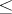is the lattice order) implies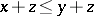for all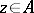and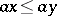for all positive real numbers. Ifis also an algebra and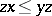and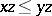for all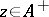, the positive cone of, thenis called an-algebra (a lattice-ordered algebra, Riesz algebra).
A Riesz algebrais called an-algebra (for "function" ) whenever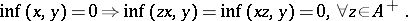An important example of an-algebra is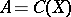, the space of continuous functions (cf. Continuous functions, space of) on some topological space. Other examples are spaces of Baire functions, measurable functions and essentially bounded functions.-Algebras play an important role in operator theory. The second commutant of a commuting subset of bounded Hermitian operators on some Hilbert space is an-algebra. A linear operatoron some vector latticeis called an orthomorphism wheneveris the difference of two positive orthomorphisms; a positive orthomorphismonleaves the positive cone ofinvariant and satisfies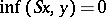whenever. The space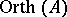of all orthomorphisms ofis an important example of an-algebra in the theory of vector lattices.
A vector latticeis termed Archimedean if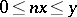(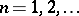) implies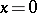. Archimedean-algebras are automatically commutative and associative. An Archimedean-algebra with unit element is semi-prime (i.e., the only nilpotent element is). The latter two properties are nice examples of the interplay between order properties and algebraic properties in an-algebra. Many properties ofare inherited by an-algebrawith a unit element (under some additional completeness condition), such as the existence of the square root of a positive element (if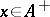, then there exists a unique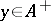such that) and the existence of an inverse: ifis the unit element ofand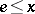, then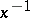exists in.